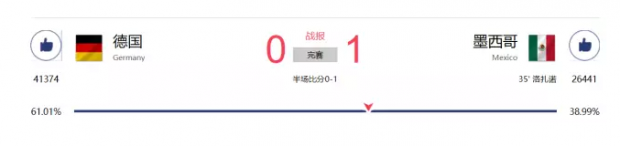A胜：下注338元

B胜：下注39元xa-(x+y+z)
yb-(x+y+z)
zc-(x+y+z)

xa-(x+y+z)≥0
yb-(x+y+z)≥0
zc-(x+y+z)≥0

(x+y+z)/a≤x
(x+y+z)/b≤y
(x+y+z)/c≤z

(x+y+z)(1/a+1/b+1/c)≤x+y+z

037篇文章 1次访问 1年前更新

• 文章归档
• 最新文章